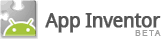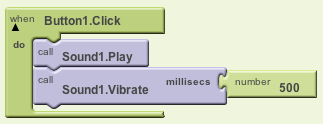## CPSC 110-08: Computing on Mobile Phones Spring 2012 Number Conversion Algorithms

### CS Principles

This activity introduces the concept that abstractions built upon binary sequences can be used to represent all digital data . It also introduces the concept of an algorithm. It focuses on the following learning objectives:
• 5b. Explanation of how number bases, including binary and decimal, are used for reasoning about digital data.
• 16a. Use of natural language, pseudo-code, or a visual or textual programming language to express an algorithm.

### Introduction

This lesson assumes you have completed the homework on binary and hexadecimal number systems. That homework decribed how the binary, decimal, and hexadecimal number systems work and showed how to convert from one number system to another.

In this lesson we want generalize what we learned their by seeing those number systems as specific examples of a more general concept, a positional number system.

We will develop algorithms that will enable you to perform conversions from one number system to another.

The type of generalization we are doing in this lesson is another example of the abstraction principle in computer science -- here we are focusing on a general pattern that holds true for all positional number systems.

### Algorithms and Pseudocode

An algorithm is a step-by-step procedure to perform some computation. For example, the steps you take in the Hello Purr app when the button is clicked is an example of a simple 2-step algorithm:To help us talk about algorithms we will use pseudocode, a language or notation that has many of the structures of a programming language but is easy to read. Pseudocdes are halfway between natural languages like English and formal programming languages.

### Positional Number Systems

Let's review some of the key points that you learned in the Khan Academy videos.

• Our decimal number system (and the binary and hexadecimal systems) are particular instances of the more general concept of a positional number system.

• In a positional number system the same symbol can represent different values depending on its position (or place) in the numeral. For example, in 91, the 9 represents 90 (the 10s place) but in 19 it represents 9 (the ones place). Contrast this with how symbols work in a non-positional system, like Roman numerals, where X always represents 10.

• The base of a number system represents the number of symbols it has:  Name Symbols Base Decimal 10 0, 1, 2, 3, 4, 5, 6, 7, 8, 9 Binary 2 0, 1 Hexadecimal 16 0, 1, 2, 3, 4, 5, 6, 7, 8, 9, A, B, C, D, E, F Octal 8 0, 1, 2, 3, 4, 5, 6, 7

• Positional number systems use exponentiation to determine a symbol's value based on its place. We can use this idea to convert from any system into the decimal system:
SystemBase ValueConversion Formula Decimal Value
Decimal10 104 (1 × 102) + (0 × 101) + (4 × 100) 100 + 0 + 4 = 104
Binary2 111 (1 × 22) + (1 × 21) + (1 × 20) 4 + 2 + 1 = 7
Octal8 104 (1 × 82) + (0 × 81) + (4 × 80) 64 + 0 + 4 = 68
Hexadecimal16 FEC (F × 162) + (E × 161) + (C × 80) 15 × 256 + 14 × 16 + 12 × 1 = 3840 + 224 + 12 = 4076

### Conversion Algorithms

Let's summarize these conversion formulas by developing an general algorithm that will convert from any base into decimal.

### Algorithm to Convert From Any Base to Base 10 Decimal

1. Let n be the number of digits in the number. For example, 104 has 3 digits, so n=3.
2. Let b be the base of the number. For example, 104 is decimal so b = 10.
3. Let s be a running total, initially 0.
4. For each digit in the number, working left to right do:
Subtract 1 from n.
Multiply the digit times bn and add it to s.
5. When your done with all the digits in the number, its decimal value will be s

Let's try it on the binary number 1011.

```Let n = 4.
Let b = 2.
Let s = 0.
First digit,  1: n = 3, 1 × bn is 1 × 23 = 8. So s = 8.
Second digit, 0: n = 2, 0 × bn is 0 × 22 = 0. So s = 8.
Third digit,  1: n = 1, 1 × bn is 1 × 21 = 2. So s = 10
Last digit,   1: n = 0, 1 × bn is 1 × 20 = 1. So 10112 = 1110
```
Digit n Value = Digit * bnRunning Total
131 × 23 = 88
020 × 22 = 08
111 × 21 = 210
101 × 20 = 111

Let's try it on the hex number 7E.

```Let n = 2.
Let b = 16.
Let s = 0.
First digit, 7: n = 1, 7 × bn is 7 × 161 = 7 × 16 = 112. So s = 112.
Last digit,  E: n = 0, 14 × bn is 14 × 160 = 14. So s = 112 + 14 = 126. So 7E16 = 12610
```
Digit n Value = Digit * bnRunning Total
717 × 161 = 112114
E014 × 160 = 14126

Let's try it on the octal number 124.

```Let n = 3.
Let b = 8.
Let s = 0.
First digit,  1: n = 2, 1 × bn is 1 × 82 = 1 × 64 = 64. So s = 64.
Second digit, 2: n = 1, 2 × bn is 2 × 81 = 2 × 8 = 16. So s = 64 + 16 = 80.
Last digit,   4: n = 0, 4 × bn is 4 × 80 = 4. So s = 80 + 4 = 84. So 1248 = 8410
```
Digit n Value = Digit * bnRunning Total
121 × 82 = 6464
212 × 81 = 1680
404 × 80 = 484

### Algorithm to Convert From Decimal To Another Base

1. Let n be the decimal number.
2. Let m be the number, initially empty, that we are converting to. We'll be composing it right to left.
3. Let b be the base of the number we are converting to.
4. Repeat until n becomes 0
Divide n by b, letting the result be d and the remainder be r.
Write the remainder, r, as the leftmost digit of b.
Let d be the new value of n.

Let's use the algorithm to convert 45 into binary.

```Let n = 45.
Let b = 2.
Repeat
45 divided by b is 45/2 = 22 remainder 1. So d=22 and r=1. So m=     1 and the new n is 22.
22 divided by b is 22/2 = 11 remainder 0. So d=11 and r=1. So m=    01 and the new n is 11.
11 divided by b is 11/2 =  5 remainder 1. So d=5  and r=1. So m=   101 and the new n is 5.
5 divided by b is 5/2  =  2 remainder 1. So d=2  and r=1. So m=  1101 and the new n is 2.
2 divided by b is 2/2  =  1 remainder 0. So d=1  and r=0. So m= 01101 and the new n is 1.
1 divided by b is 1/2  =  0 remainder 1. So d=0  and r=1. So m=101101 and the new n is 0. So 4510 = 1011012
```

Let's use it to convert 99 into binary.

```Let n = 99.
Let b = 2.
Repeat
99 divided by b is 99/2 = 49 remainder 1. So d=49 and r=1. So m=      1 and the new n is 49.
49 divided by b is 49/2 = 24 remainder 1. So d=24 and r=1. So m=     11 and the new n is 24.
24 divided by b is 24/2 = 12 remainder 0. So d=12 and r=0. So m=    011 and the new n is 12.
12 divided by b is 12/2 =  6 remainder 0. So d=6  and r=0. So m=   0011 and the new n is 6.
6 divided by b is  6/2 =  3 remainder 0. So d=3  and r=0. So m=  00011 and the new n is 3.
3 divided by b is  3/2 =  1 remainder 1. So d=1  and r=1. So m= 100011 and the new n is 1.
1 divided by b is  1/2 =  0 remainder 1. So d=0  and r=1. So m=1100011 and the new n is 0.  So 9910 = 11000112

```

Let's use it to convert 45 into hexadecimal.

```Let n = 45.
Let b = 16.
Repeat
45 divided by b is 45/16 = 2 remainder 13. So d=2 and r=13. So m= D and the new n is 2.
2 divided by b is  2/16 = 0 remainder  2. So d=0 and r=2.  So m=2D and the new n is 0. So 4510 = 2D16.
```

Let's use it to convert 99 into hexadecimal.

```Let n = 99.
Let b = 16.
Repeat
99 divided by b is 99/16 = 6 remainder 3. So d=6 and r=3. So m= 3 and the new n is 6.
6 divided by b is  6/16 = 0 remainder 6. So d=0 and r=6. So m=63 and the new n is 0. So 9910 is 6316.
```

### In-class Exercises

1. Convert the following numbers to decimal notation.
1. The binary number 111.
2. The binary number 1011.
3. The binary number 1011 1011.
4. The hex number 61.
5. The hex number DA.
6. The hex number FEE.
2. Convert the following decimal numbers as indicated.
1. Convert decimal 12 to binary.
2. Convert decimal 44 to binary.
3. Convert decimal 254 to hex.
4. Convert decimal 16 to hex.
3. Challenge: Convert decimal 125 to octal (base 8) notation.